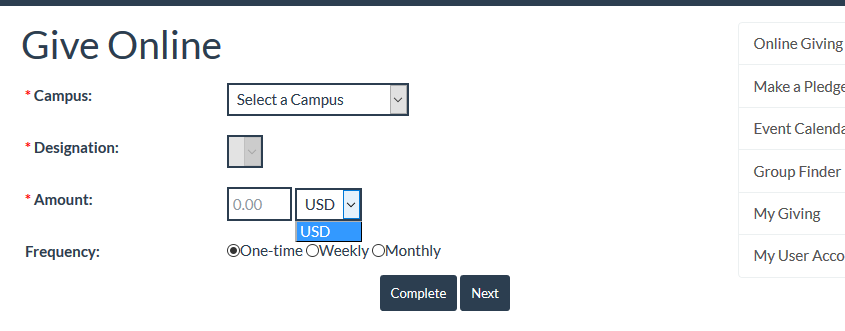## Currencies

This Resource File controls the list of available currencies when accepting payment and can be edited using the Portal Admin page.#### Sample Currencies File

``````<?xml version="1.0" encoding="utf-8" ?>
<currencies>
<currency name="US Dollars" value="USD" code="840" />
<!--  <currency name="Philippine Pesos" value="PHP" code="608" />
<currency name="HKD" value="HKD" code="344" />
<currency name="SGDs" value="SGD" code="702" />
<currency name="CNY" value="CNY" code="156" />
<currency name="JPY" value="JPY" code="392" />
<currency name="TWD" value="TWD" code="901" />
<currency name="AUD" value="AUD" code="036" />
<currency name="EUR" value="EUR" code="978" />
<currency name="GBP" value="GBP" code="826" />